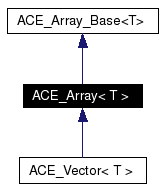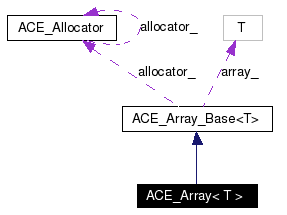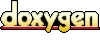# ACE_Array< T > Class Template Reference

A dynamic array class. More...

`#include <Containers_T.h>`

Inheritance diagram for ACE_Array< T >:[legend]
Collaboration diagram for ACE_Array< T >:[legend]
List of all members.

## Public Types

typedef T TYPE
typedef ACE_Array_Iterator<
T >
ITERATOR

## Public Member Functions

ACE_Array (size_t size=0, ACE_Allocator *alloc=0)
Dynamically create an uninitialized array.

ACE_Array (size_t size, const T &default_value, ACE_Allocator *alloc=0)
Dynamically initialize the entire array to the {default_value}.

ACE_Array (const ACE_Array< T > &s)
Copy constructor.

void operator= (const ACE_Array< T > &s)
Assignment operator.

bool operator== (const ACE_Array< T > &s) const
Equality comparison operator.

bool operator!= (const ACE_Array< T > &s) const
Inequality comparison operator.

## Detailed Description

### template<class T> class ACE_Array< T >

A dynamic array class.

This class extends ACE_Array_Base, adding comparison operators.

Requirements and Performance Characteristics

• Internal Structure Dynamic array
• Duplicates allowed? Yes
• Random access allowed? Yes
• Search speed N/A
• Insert/replace speed O(1)
• Iterator still valid after change to container?
• In general, yes.
• If array size is changed during iteration, no.
• Frees memory for removed elements? No
• Items inserted by Value
• Requirements for contained type
1. Default constructor
2. Copy constructor
3. operator=
4. operator!=

ACE_Array_Base. This class inherits its operations and requirements.

Definition at line 1944 of file Containers_T.h.

## Member Typedef Documentation

 template typedef ACE_Array_Iterator ACE_Array< T >::ITERATOR
 Reimplemented from ACE_Array_Base< T >. Definition at line 1950 of file Containers_T.h.

 template typedef T ACE_Array< T >::TYPE
 Reimplemented from ACE_Array_Base< T >. Definition at line 1948 of file Containers_T.h.

## Constructor & Destructor Documentation

 template ACE_INLINE ACE_Array< T >::ACE_Array ( size_t size = 0, ACE_Allocator * alloc = 0 )
 Dynamically create an uninitialized array. Initialize an empty array of the specified size using the provided allocation strategy. Definition at line 272 of file Containers_T.inl. ```00274 : ACE_Array_Base (size, alloc) 00275 { 00276 } ```

 template ACE_INLINE ACE_Array< T >::ACE_Array ( size_t size, const T & default_value, ACE_Allocator * alloc = 0 )
 Dynamically initialize the entire array to the {default_value}. Initialize an array the given size placing the default_value in each index. Definition at line 279 of file Containers_T.inl. ```00282 : ACE_Array_Base (size, default_value, alloc) 00283 { 00284 } ```

 template ACE_INLINE ACE_Array< T >::ACE_Array ( const ACE_Array< T > & s )
 Copy constructor. The copy constructor performs initialization by making an exact copy of the contents of parameter {s}, i.e., *this == s will return true. Definition at line 289 of file Containers_T.inl. ```00290 : ACE_Array_Base (s) 00291 { 00292 } ```

## Member Function Documentation

 template ACE_INLINE bool ACE_Array< T >::operator!= ( const ACE_Array< T > & s ) const
 Inequality comparison operator. Compare this array with {s} for inequality such that {*this} != {s} is always the complement of the boolean return value of {*this} == {s}. Definition at line 308 of file Containers_T.inl. ```00309 { 00310 return !(*this == s); 00311 } ```

 template ACE_INLINE void ACE_Array< T >::operator= ( const ACE_Array< T > & s )
 Assignment operator. Assignment operator performs an assignment by making an exact copy of the contents of parameter {s}, i.e., *this == s will return true. Note that if the {max_size_} of {array_} is >= than {s.max_size_} we can copy it without reallocating. However, if {max_size_} is < {s.max_size_} we must delete the {array_}, reallocate a new {array_}, and then copy the contents of {s}. Definition at line 297 of file Containers_T.inl. References ACE_Array_Base< T >::operator=(). ```00298 { 00299 // Check for "self-assignment". 00300 00301 if (this != &s) 00302 this->ACE_Array_Base::operator= (s); 00303 } ```

 template bool ACE_Array< T >::operator== ( const ACE_Array< T > & s ) const
 Equality comparison operator. Compare this array with {s} for equality. Two arrays are equal if their {size}'s are equal and all the elements from 0 .. {size} are equal. Definition at line 1918 of file Containers_T.cpp. References ACE_Array_Base< T >::size(). ```01919 { 01920 if (this == &s) 01921 return true; 01922 else if (this->size () != s.size ()) 01923 return false; 01924 01925 const size_t len = s.size (); 01926 for (size_t slot = 0; slot < len; ++slot) 01927 if ((*this)[slot] != s[slot]) 01928 return false; 01929 01930 return true; 01931 } ```

The documentation for this class was generated from the following files:
Generated on Thu Nov 9 11:19:13 2006 for ACE by1.3.6